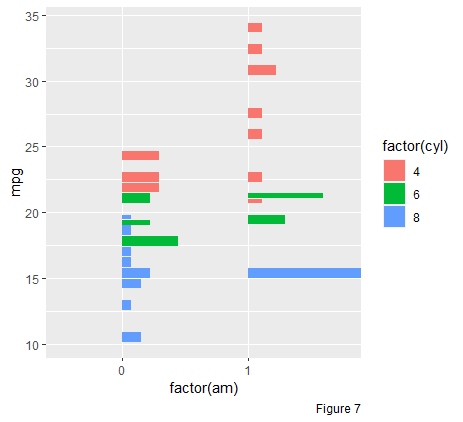# Histogram and Density

#### 2021-03-10

Histograms (and bar plots) are common tools to visualize a single variable. The x axis is often used to locate the bins and the y axis is for the counts. Density plots can be considered as the smoothed version of the histogram.

Boxplot is another method to visualize one dimensional data. Five summary statistics can be easily traced on the plot. However, compared with histograms and density plots, boxplot can accommodate two variables, groups (often on the x axis) and ys (on the y axis).

In ggplot2, geom_histogram and geom_density only accept one variable, x or y (swapped). Providing both positions is forbidden. Inspired by the boxplot (geom_boxplot in ggplot2), we create functions geom_histogram_, geom_bar_ and geom_density_ which can accommodate both variables, just like the geom_boxplot!

## Hist (histogram and bar plot)

### Two dimensional bar plot: geom_bar_

Consider the mtcars data set and suppose that we are interested in the relationship of number of gears given the cyl (number of cylinders).

ggplot(mtcars,
mapping = aes(x = factor(cyl), y = factor(gear))) +
geom_bar_() +
labs(caption = "Figure 1")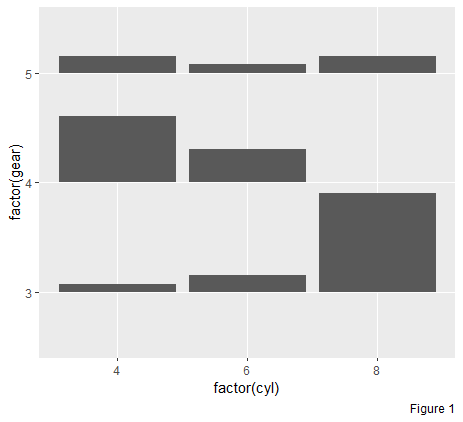Though the Figure 1, we can tell that

• Compare vertically: given the number of engines, tell the gears

• Most V8 engine cars prefer 3 gear transmission. V8 cars do not use 4 gear transmission

• Most V4 engine cars prefer 4 gears transmission.

• Compare horizontally: given the number of gears, tell the engines

• Most 3 gear transmission cars carry a V8 engine.

• Most 4 gear transmission cars carry a V4 engine, then V6 engine, but never V8 engine.

• Five gear transmission cars can carry either a V4, V6 or V8 engine. However, compared with other two transmissions, 5 gear is not a common choice.

### Two dimensional histogram: geom_histogram_

Suppose now, we are interested in the distribution of mpg (miles per gallon) with the respect to the cyl (as “x” axis) and gear (as “fill”)

g <- ggplot(mtcars,
mapping = aes(x = factor(cyl), y = mpg, fill = factor(gear))) +
geom_histogram_() +
labs(caption = "Figure 2")
g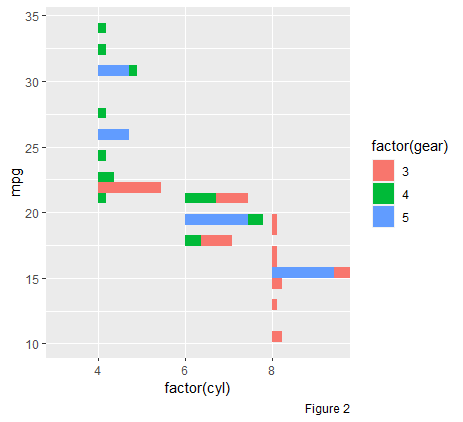Through the Figure 2, we can easily tell that as the number of cylinders rises, the miles/gallon drops significantly. Moreover, the number of six cylinder cars is much less that the other two in our data. In addition, the transmission of V8 cars is either 3 or 5 (identical to the conclusion we draw before).

### Just call geom_hist!

Function geom_histogram_ is often used as one factor is discrete and the other is continuous, while function geom_bar_ accommodate two discrete variables. The former one relies on stat = bin_ and the latter one is on stat = count_. However, if we turn the factor of interest as numerical in geom_bar_, there would be no difference between the output of a bar plot and a histogram. Hence, function geom_hist is created by simplifying the process. It understands both cases and users can just call geom_hist to create either a bar plot or a histogram.

## Density

We could also draw density plot side by side to better convey the data of interest. With geom_density_, both summaries can be displayed simultaneously in one chart.

g +
# parameter "positive" controls where the summaries face to
geom_density_(positive = FALSE, alpha = 0.2) +
labs(caption = "Figure 3")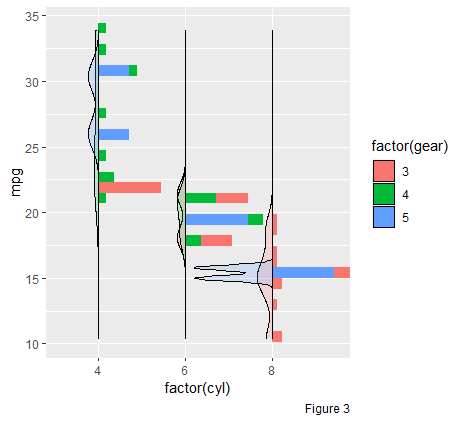Parameter scaleY is often used to set the scales of each density (or bar). The default “data” indicates that the area of each density is proportional to the count of such group.

cyl count
4 11
6 7
8 14

The area of group cylinder 8 is approximately twice as much as the group cylinder 6.

If only one variable is provided in geom_density_() (so does geom_histogram_() and geom_bar_()), the original function geom_density() will be executed automatically.

ggplot(mtcars,
mapping = aes(x = mpg, fill = factor(cyl))) +
geom_density_(alpha = 0.3) +
labs(caption = "Figure 4")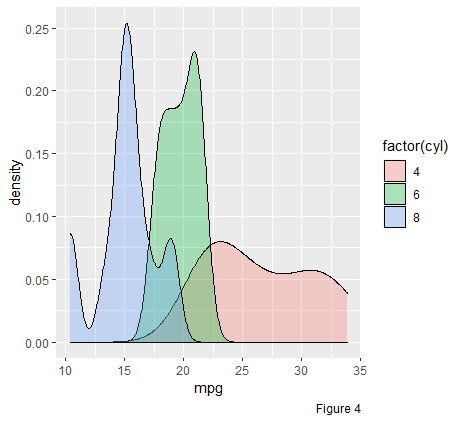which is identical to call function geom_density(). However, if we take a look at this chart, we can realize that the area for each group is 1. In other words, the whole area is 3 in total. In geom_density_, we have a parameter called asOne. If it is set as TRUE, the sum of the density area is 1 and the area for each group is proportional to its own count.

ggplot(mtcars,
mapping = aes(x = mpg, fill = factor(cyl))) +
geom_density_(as.mix = TRUE, alpha = 0.3) +
labs(caption = "Figure 5")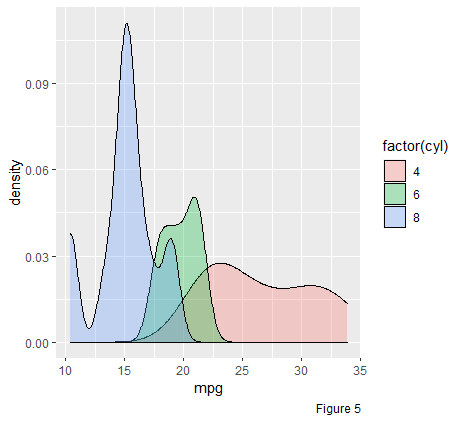## Set Positions

Note that when we set position in function geom_histogram_() or geom_density, we should use the underscore case, that is “stack_”, “dodge_” or “dodge2_” (instead of “stack”, “dodge” or “dodge2”).

### Position stack_

Similar to geom_density, we can stack the density on top of each other by setting position = 'stack_' (default position = 'identity_')

ggplot(mtcars,
mapping = aes(x = factor(am), y = mpg, fill = factor(cyl))) +
geom_density_(position = "stack_",
adjust = 0.75,
as.mix = TRUE) +
labs(caption = "Figure 6")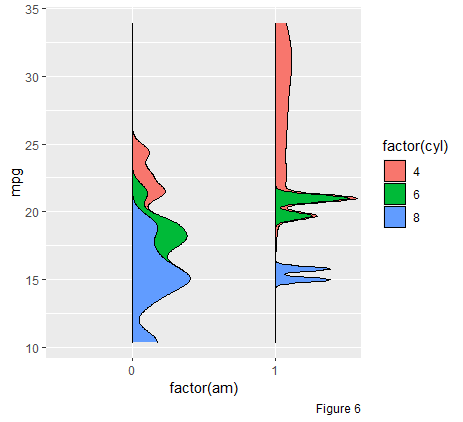### Position dodge_(dodge2_)

Dodging preserves the vertical position of an geom while adjusting the horizontal position (the default position of geom_hist_, geom_histogram_ and geom_bar_ is stack_)

ggplot(mtcars,
mapping = aes(x = factor(am), y = mpg, fill = factor(cyl))) +
# use more general function geom_hist_
# dodge2 works without a grouping variable in a layer
geom_hist_(position = "dodge2_") +
labs(caption = "Figure 7")